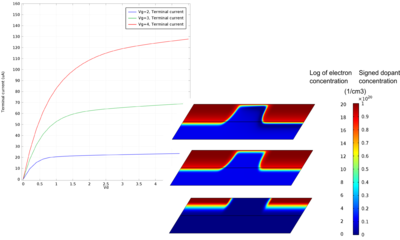# Galleria delle Applicazioni

## DC Characteristics of a MOS Transistor (MOSFET)

Application ID: 14609

This model calculates the DC characteristics of a simple MOSFET. The drain current versus gate voltage characteristics are first computed in order to determine the threshold voltage for the device. Then the drain current vs drain voltage characteristics are computed for several gate voltages. The linear and saturation regions for the device can be identified from these plots.This model example illustrates applications of this type that would nominally be built using the following products: